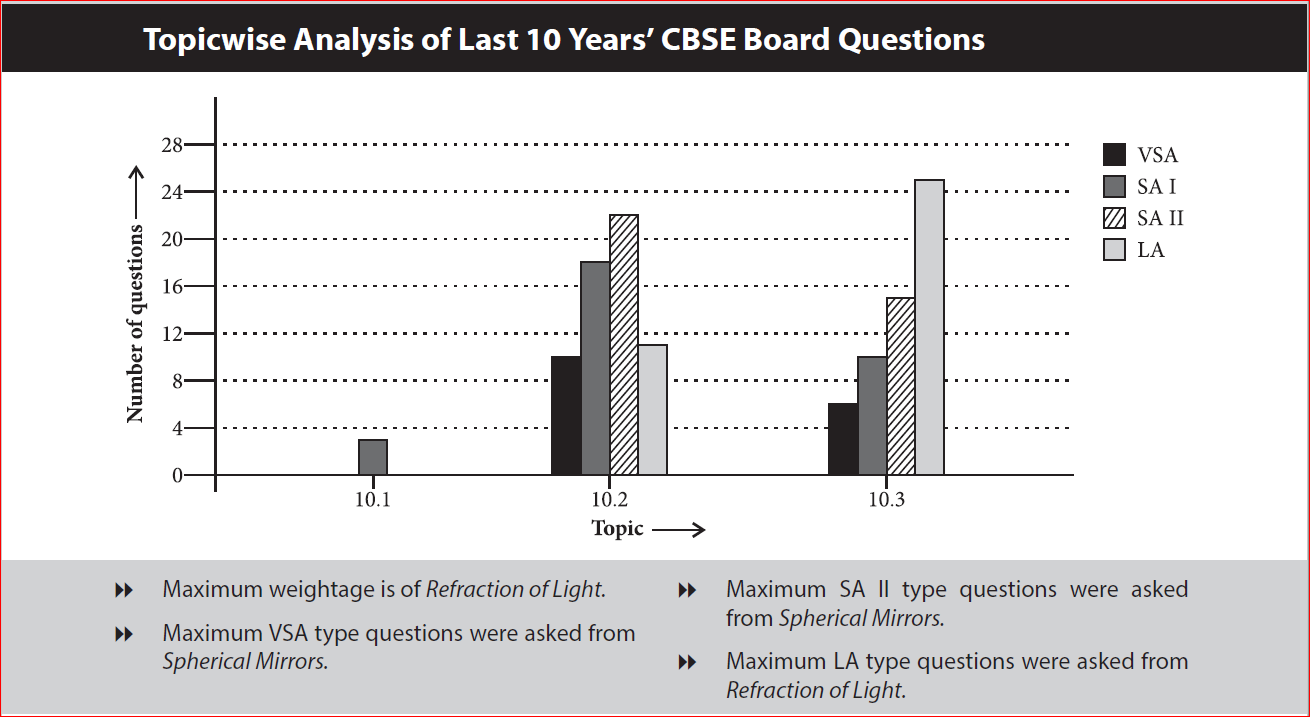# NCERT Solutions for Class 10 Science Chapter 10: Light Reflection and Refraction

### NCERT Solutions for class 10 Chapter 10 – Light – Reflection and Refraction### NCERT Solutions For Class 10 Science Chapter 10 – Light Reflection and Refraction

Chapter 10 Light Reflection and Refraction is one of the important chapters in class 10 science and the expected marks weightage of the chapter according to the latest marking scheme is 7 marks. In chapter 10 of class 10 science, students will get well versed with light phenomena such as refraction and reflection. One will learn about spherical mirrors, image formation and to draw ray diagrams.

Topics covered in this chapter:

• Reflection of Light
• Spherical Mirrors
• Image Formation by Spherical Mirrors
• Representation of Images Formed by Spherical Mirrors Using Ray Diagram – 4 Questions ( 2 short, 2 long)
• Mirror Formula and Magnification – 2 Questions (2 Numerical)
• Refraction of Light – 5 Questions ( 1 numerical, 2 Long answers and 1 Short answer)
• Power of Lens – 3 Questions ( 2 Short answers and 1 numerical)

Have you ever wondered what makes things visible to us? The answer to the question is light. During the day, it is sunlight that helps us see objects. When light falls on an object, it reflects it. This reflected ray when received by our eyes helps us see things. There are numerous wonderful phenomena that are associated with light such as the formation of the rainbow, of stars and many more. In chapter 10 Light Reflection and Refraction of class 10 Science, let us study the phenomena of refraction and reflection using straight-line propagation of light. We shall also try to understand the reflection of light by spherical mirrors in this chapter.

NCERT Solutions For Class 10 Science Chapter 10 Light Reflection and Refraction: In this Chapter candidates can find light reflection and refraction class 10 NCERT solutions. Working on the light chapter of class 10 NCERT solutions will help candidates to build a strong foundation over the subject Physics. Knowing light reflection and refraction class 10 questions and answers will help students of class 10 to bag a decent score in class 10 board exams as well.

Along with NCERT Solutions For Class 10 Science Chapter 10 Light Reflection and Refraction candidates can also find light reflection and refraction class 10 numericals questions in this article. Go through them will help candidates get a clear idea about how to approach the problems which in turn helps you to solve them in the most efficient way.

### NCERT Solutions for Class 10 Science Chapter 10 Light Reflection and Refraction

Subtopics under NCERT class 10 science book activities solutions chapter 10:

1. Light – Reflection And Refraction
2. Reflection Of Light
3. Spherical Mirrors
4. Refraction Of Light
Scroll to Top